# Quantum Chemistry Quantum Mechanics Postulates Timedependent Schrdinger Equation

• Slides: 22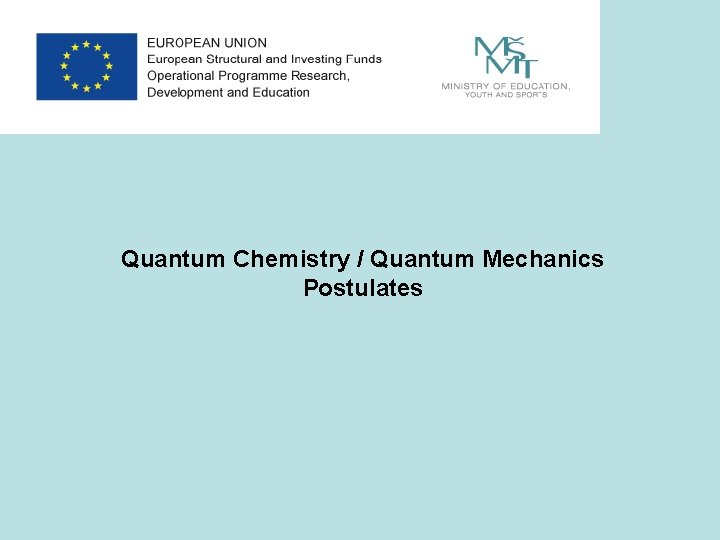Quantum Chemistry / Quantum Mechanics Postulates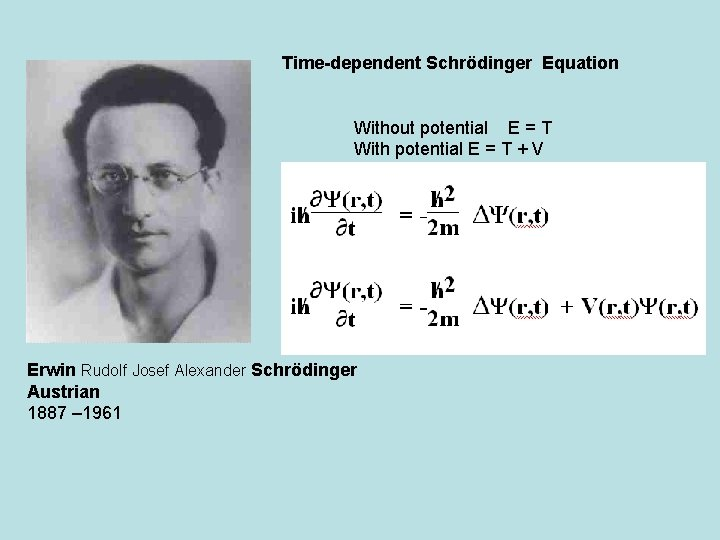Time-dependent Schrödinger Equation Without potential E = T With potential E = T + V Erwin Rudolf Josef Alexander Schrödinger Austrian 1887 – 1961Postulates of Quantum Mechanics Postulate 1: The state of a quantum mechanical system is completely specified by a wave function ψ (r, t) that depends on the coordinates of the particles (r) and time t. These functions are called wave functions or state functions. For 2 particle system: Wave function contains all the information about a system. wave function classical trajectory (Quantum mechanics) (Newtonian mechanics) Meaning of wave function: P(r) = |ψ|2 = => the probability that the particle can be found at a particular point x and a particular time t. (Born’s / Copenhagen interpretation)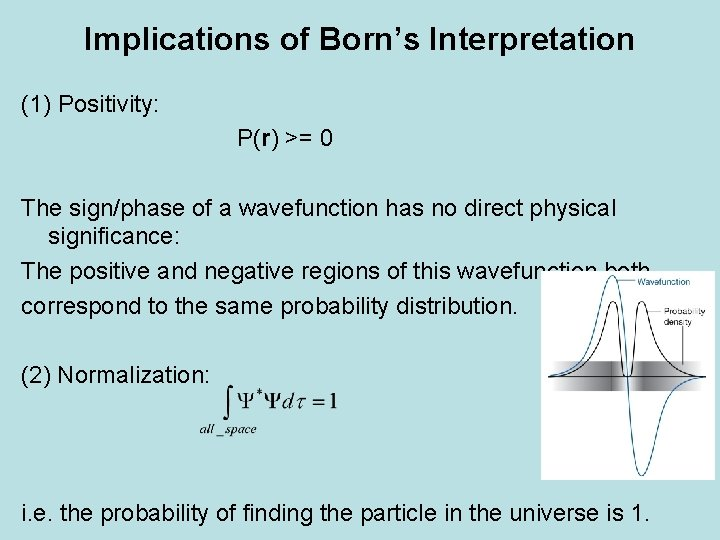Implications of Born’s Interpretation (1) Positivity: P(r) >= 0 The sign/phase of a wavefunction has no direct physical significance: The positive and negative regions of this wavefunction both correspond to the same probability distribution. (2) Normalization: i. e. the probability of finding the particle in the universe is 1.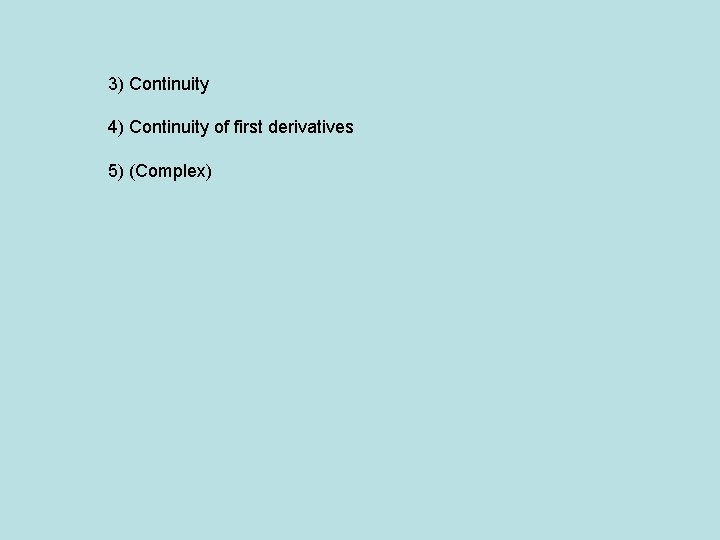3) Continuity 4) Continuity of first derivatives 5) (Complex)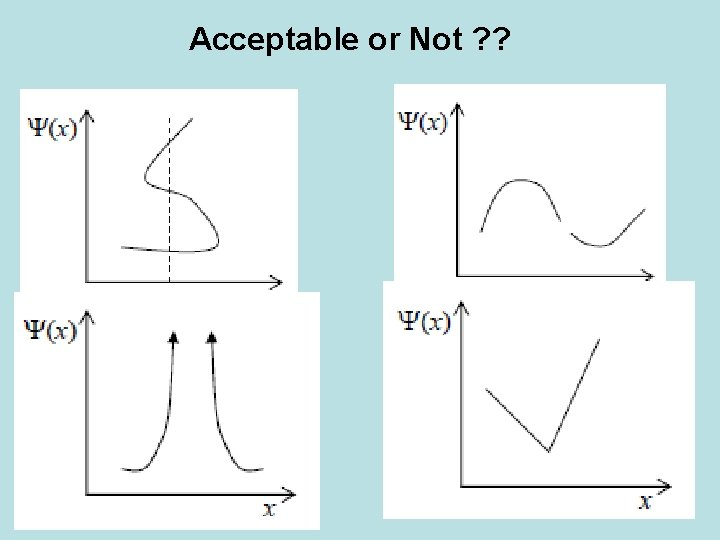Acceptable or Not ? ?Postulate 2 To every physical property, observable in classical mechanics, there corresponds a linear, hermitian operator in quantum mechanics.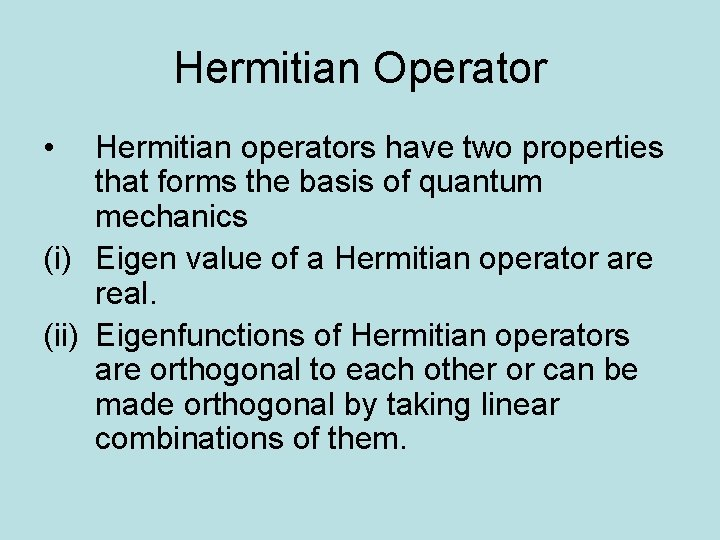Hermitian Operator • Hermitian operators have two properties that forms the basis of quantum mechanics (i) Eigen value of a Hermitian operator are real. (ii) Eigenfunctions of Hermitian operators are orthogonal to each other or can be made orthogonal by taking linear combinations of them.Identifying the operatorsHermitian operator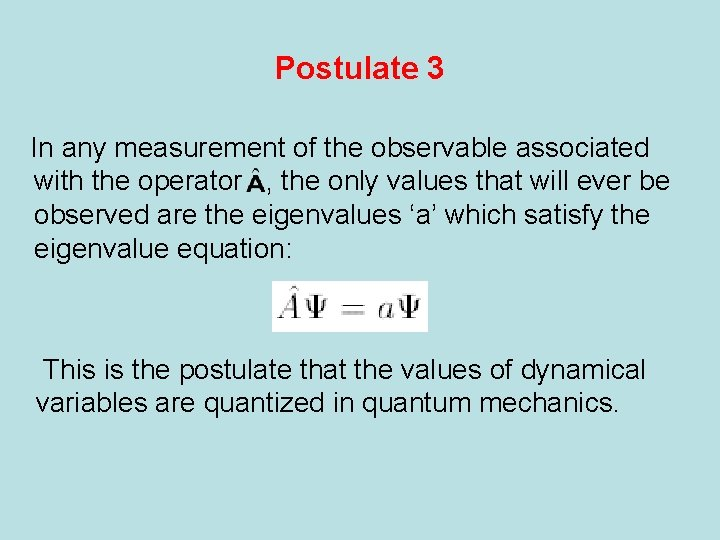Postulate 3 In any measurement of the observable associated with the operator , the only values that will ever be observed are the eigenvalues ‘a’ which satisfy the eigenvalue equation: This is the postulate that the values of dynamical variables are quantized in quantum mechanics.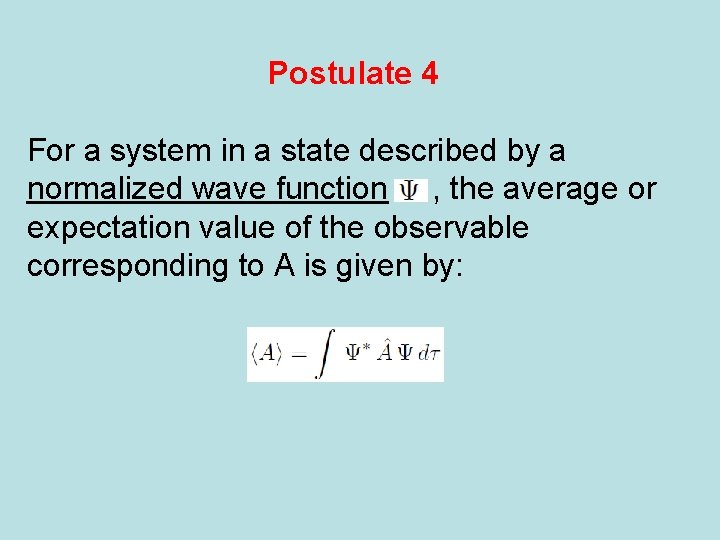Postulate 4 For a system in a state described by a normalized wave function , the average or expectation value of the observable corresponding to A is given by:Add 4: Correspondence principle 1913/1920 For every physical quantity one can define an operator. The definition uses formulae from classical physics replacing quantities involved by the corresponding operators Niels Henrik David Bohr Danish 1885 -1962 QM is then built from classical physics in spite of demonstrating its limitsPostulate 5 The wave function of a system evolves in time in accordance with the time dependent Schrödinger equation: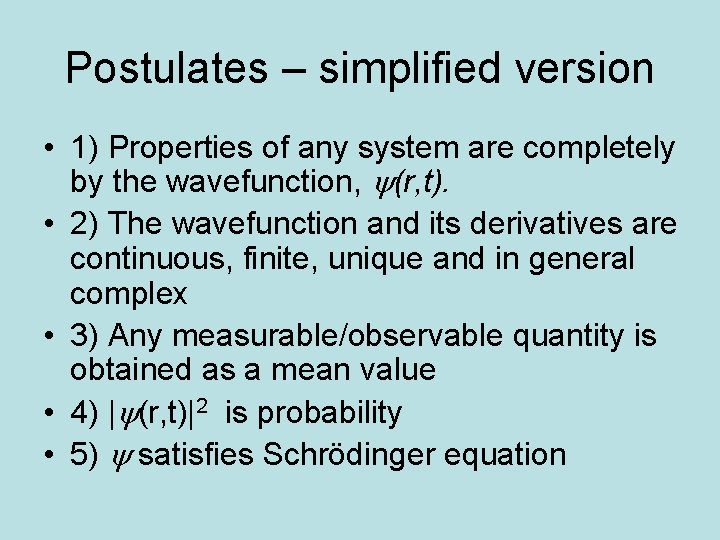Postulates – simplified version • 1) Properties of any system are completely by the wavefunction, (r, t). • 2) The wavefunction and its derivatives are continuous, finite, unique and in general complex • 3) Any measurable/observable quantity is obtained as a mean value • 4) | (r, t)|2 is probability • 5) satisfies Schrödinger equation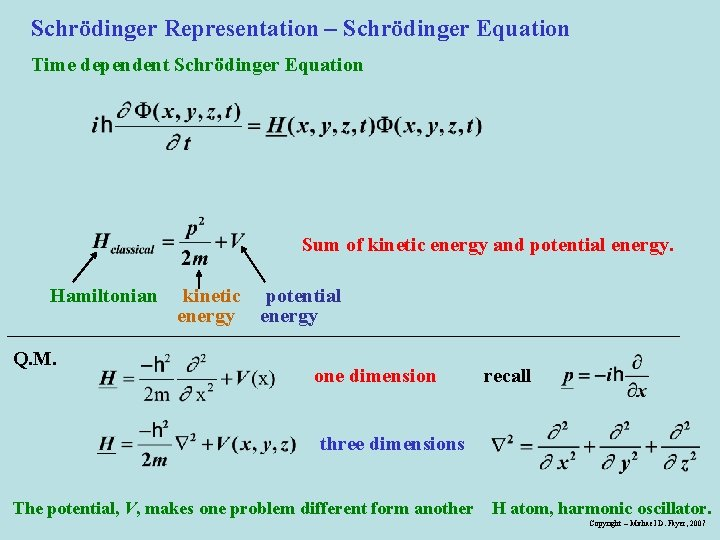Schrödinger Representation – Schrödinger Equation Time dependent Schrödinger Equation Sum of kinetic energy and potential energy. Hamiltonian Q. M. kinetic potential energy one dimension recall three dimensions The potential, V, makes one problem different form another H atom, harmonic oscillator. Copyright – Michael D. Fayer, 2007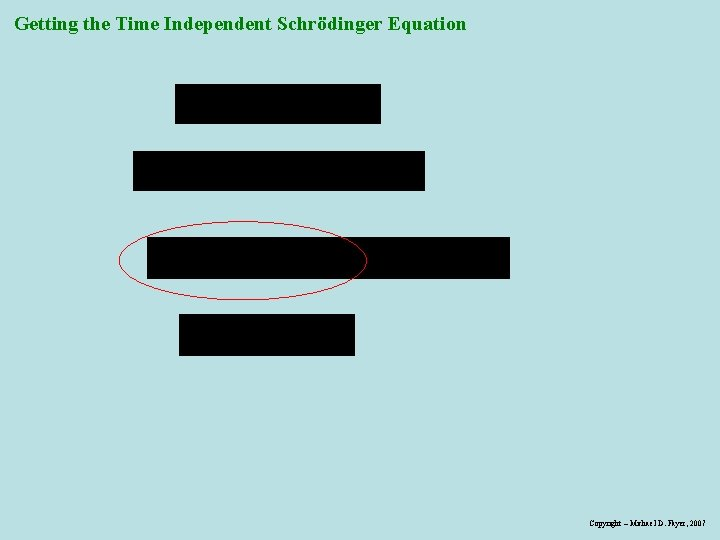Getting the Time Independent Schrödinger Equation Copyright – Michael D. Fayer, 2007Kinetic energy Classical quantum operator In 3 D : Calling the laplacian Pierre Simon, Marquis de Laplace (1749 -1827)Chemistry is nothing but an application of Schrödinger Equation (Dirac) < YI Y> <Y IOI Y > Dirac notations Paul Adrien Dirac 1902 – 1984 Dirac’s mother was British and his father was Swiss.Uncertainty principle the Heisenberg uncertainty principle states that locating a particle in a small region of space makes the momentum of the particle uncertain; and conversely, that measuring the momentum of a particle precisely makes the position uncertain We already have seen incompatible operators Werner Heisenberg German 1901 -1976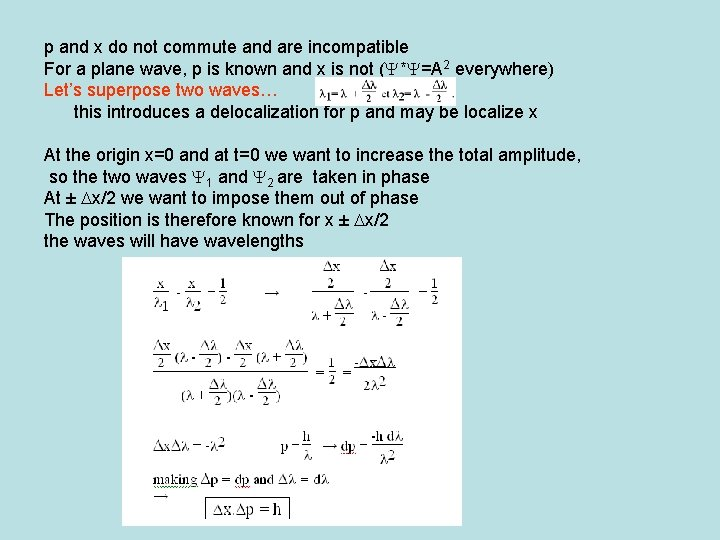p and x do not commute and are incompatible For a plane wave, p is known and x is not (Y*Y=A 2 everywhere) Let’s superpose two waves… this introduces a delocalization for p and may be localize x At the origin x=0 and at t=0 we want to increase the total amplitude, so the two waves Y 1 and Y 2 are taken in phase At ± Dx/2 we want to impose them out of phase The position is therefore known for x ± Dx/2 the waves will have wavelengths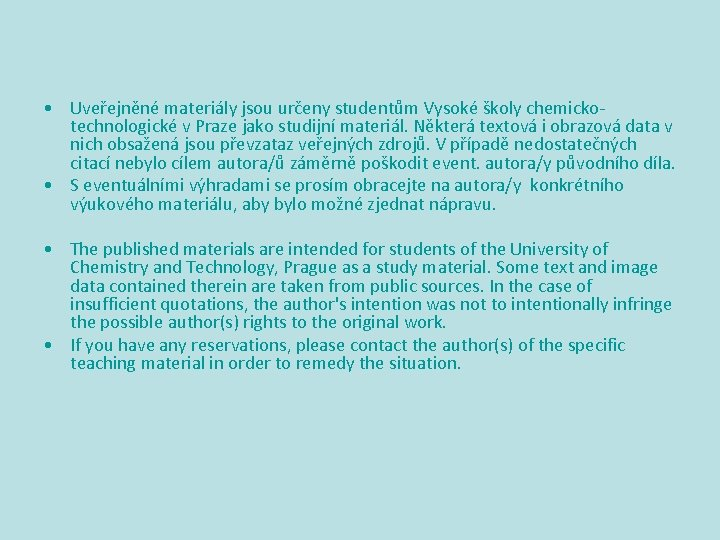• Uveřejněné materiály jsou určeny studentům Vysoké školy chemickotechnologické v Praze jako studijní materiál. Některá textová i obrazová data v nich obsažená jsou převzataz veřejných zdrojů. V případě nedostatečných citací nebylo cílem autora/ů záměrně poškodit event. autora/y původního díla. • S eventuálními výhradami se prosím obracejte na autora/y konkrétního výukového materiálu, aby bylo možné zjednat nápravu. • The published materials are intended for students of the University of Chemistry and Technology, Prague as a study material. Some text and image data contained therein are taken from public sources. In the case of insufficient quotations, the author's intention was not to intentionally infringe the possible author(s) rights to the original work. • If you have any reservations, please contact the author(s) of the specific teaching material in order to remedy the situation.